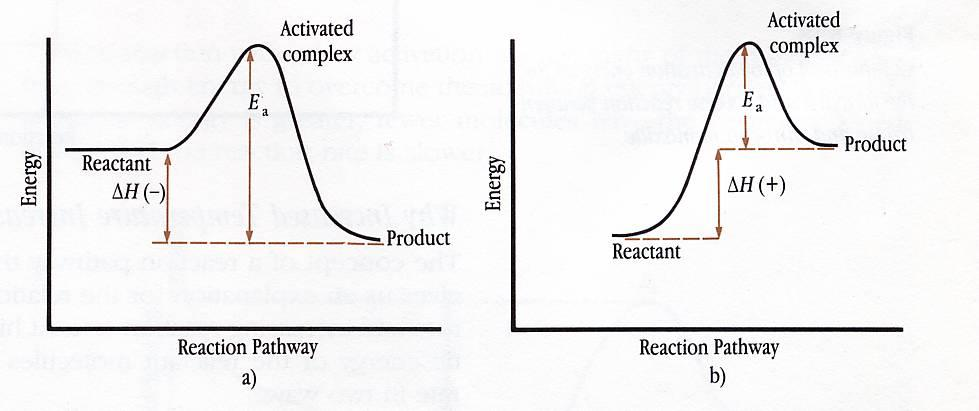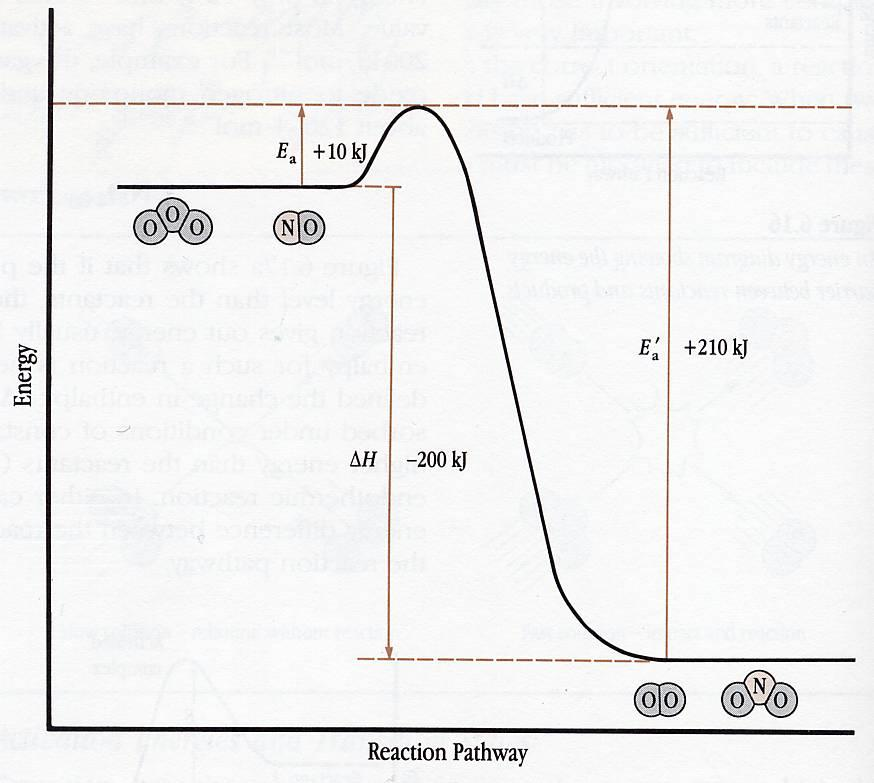# Transition State Figure 3

 Page 3/4 Date 23.04.2018 Size 404.21 Kb. #45986
1   2   3   4

### Figure 3

It shows how the energy of the reacting system changes as the reaction proceeds. The potential energy of each chemical (reactants, the activated complex, and products) is indicated along the vertical axis. The progress of the reaction is plotted along the horizontal axis or reaction pathway. All potential energy diagrams have a similar profile. The reaction passes through energy maximum, known as the transition state. It is at this highest energy point that the activated complex is formed.
The difference between the energy of the reactants in the beginning and when they are in the transition state is the activation energy or Ea. The activation energy, you will recall, is the minimum energy required for the reaction to occur and corresponds to the energy necessary to reach the transition state and form the activated complex. Most reactions have activation energies in the range of 100 to 200 KJ for every mole of reactant present.
Figure 4a shows that if the products of the reaction are at a lower energy than the reactants, the reaction is exothermic. This type of reaction gives off energy, usually in the form of heat, and this indicated by a negative enthalpy (H) value. The change in enthalpy or H is the heat released or absorbed during a reaction, under constant pressure. If the products are of higher energy than the reactants (Figure 4b), we are dealing with an endothermic reaction. In either case the activation energy is always the difference between the energy of reactants initially and the highest point on the reaction pathway.

#### Figure 4(Exothermic) (Endothermic)

The activation energy for a reverse reaction is not the same as the activation energy for its forward reaction. For example, nitrogen monoxide will react with ozone to produce nitrogen dioxide and oxygen:

NO (g) + O3 (g)  2 NO2 (g) + O2 (g)
For this reaction, the activation energy, Ea, is +10 KJ/mol, while the enthalpy change, H, is –200 KJ/mol (see Figure 5). We find that collisions between nitrogen dioxide and oxygen sometimes lead to the formation of nitrogen monoxide and ozone.
2 NO2 (g) + O2 (g)  NO (g) + O3 (g)
This reverse reaction will have an enthalpy change of +200 KJ/mol. As a result, the activation energy of the reverse reaction (E’a) will be 210 KJ/mol (10 KJ/mol + 200 KJ/mol). The activation energy of the reverse reaction is very high, indicating that the reaction will be very slow.

#### Figure 5